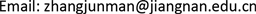1江南大学数字媒体学院，江苏 无锡

2江南大学江苏省媒体设计与软件技术重点实验室，江苏 无锡1. 引言

2. 微下击暴流仿真算法

2.1. 基于涡环的数学模型

Michael Ivan  最早根据气象观测数据提出基于涡环的微下击暴流仿真模型，其仿真结果与实测风场数据在视觉上具有较高的一致性，得到广泛应用。

{ ( x − x p ) 2 + ( y − y p ) 2 = R 2 z = z p (1)

ψ p = Γ 2π ( r max + r min ) F ( k ) (2)

{ v x = x A − x P r p v r v y = y A − y P r p v r v z = − 1 r P ∂ ( ψ I + ψ P ) ∂ r (3)

Michael Ivan模型在计算上存在较大复杂度，对特殊位置(如主涡环自身和涡环中轴线等)存在奇异性问题，需要追加计算步骤。虽然后续研究者不断提出改进方案，但在计算速度上和程序复杂度上效果并不理想。

2.2. 本文圆切线模型

Michael Ivan模型采用涡环的主要原因是微下击暴流表现出典型的涡流现象，即风场存在一个旋转中心点，而方程(2)的切线方向与涡流现象吻合。方程(2)及其切线防线的计算复杂性是Michael Ivan模型复杂度的主要来源，本节提出使用圆切线的方式大幅降低模型复杂度。

Michael Ivan模型中计算涡环切线的过程，可以由图2中圆切线近似逼近，即风场在任意一点P的风矢量的方向与圆切线一致，大小与圆心距离调整：

{ v x P = ( ( y p − y B ) ϕ ( d B ) ‖ P − O B ‖ − ( y p − y A ) ϕ ( d A ) ‖ P − O A ‖ ) V ¯ v y P = ( ( x P − x A ) ϕ ( d A ) ‖ P − O A ‖ − ( x p − x B ) ϕ ( d B ) ‖ P − O B ‖ ) V ¯ (4)

3. 基于粒子系统的风场可视化技术

3.1. 粒子系统

{ Θ k | Θ k = ( x k y k z k t k ) , k = 1 , 2 , ⋯ , N } (5)

Θ k ← ( x k + v x Θ k Δ t y k + v y Θ k Δ t z k + v z Θ k Δ t ) (6)

3.2. 拖尾技术

T 0 = Θ k T k = T k − 1 (7)

4. 仿真实验结果

5. 结论